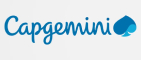New update is available. Click here to update.
Topics

# Maximum GCD

Hard0/120
Average time to solve is 40m
1 upvote## Problem statement

You are given an array 'A' of length 'N'. You can perform the following operation at most once.

``````Choose any element of the array and replace it with 'X', 1 <= 'X' <= 'M'.
``````

Return the maximum possible GCD of the array after performing the operation.

For Example:-
``````Let 'N' = 3, 'M' = 5, and 'A' = [4, 3, 2].
We can replace 3 with 2.
The maximum possible GCD is 2.
``````
Detailed explanation ( Input/output format, Notes, Images )
Constraints:-
``````1 <= 'T' <= 10
1 <= 'N' , 'M' <= 10^5
1 <= 'A[i]' <= 10^5

The Sum of 'N' overall test cases does not exceed 10^5.
Time Limit: 1 sec
``````
Sample Input 1:-
``````2
4 5
2 5 6 12
2 4
10 15
``````
Sample Output 1:-
``````2
5
``````
Explanation of sample input 1:-
``````First test case:-
We can replace 5 with 4.
The maximum possible GCD is 2.

Second test case:-
We do not need to replace any element.
The maximum possible GCD is 5.
``````
Sample Input 2:-
``````2
5 13
3 4 2 8 7
3 12
4 14 13
``````
Sample Output 2:-
``````1
2
``````Console